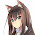## Saturday, April 19, 2014

### How the continuation monad works

I remember the first time I read the `Monad` instance for `ContT` I was so confused. I couldn't fathom how it worked because it was hard to discern the pattern.

However, I later discovered that renaming things makes the pattern much more clear:

``````import Control.Applicative

newtype ContT x m r = ContT { (>>-) :: (r -> m x) -> m x }

instance Functor (ContT x m) where
fmap f m  = ContT \$ \_return ->  -- fmap f m =
m >>- \a ->                  --     m >>= \a ->
_return (f a)                --     return (f a)

instance Applicative (ContT x m) where
pure r    = ContT \$ \_return ->  -- pure r =
_return r                    --     return r

mf <*> mx = ContT \$ \_return ->  -- mf <*> mx =
mf >>- \f ->                 --     mf >>= \f ->
mx >>- \x ->                 --     mx >>= \x ->
_return (f x)                --     return (f x)

instance Monad (ContT x m) where
return r  = ContT \$ \_return ->  -- return r =
_return r                    --     return r

m >>= f   = ContT \$ \_return ->  -- m >>= f =
m   >>- \a ->                --     m   >>= \a ->
f a >>- \b ->                --     f a >>= \b ->
_return b                    --     return b``````

1.I remember that SPJ also showed that adding some omitted function parameters to the both ends of function definition makes lens code much more understandable.
But right now I'm trying to understand the internals of pipe (yes - you've already provided great documentation and metaphors, but some time is needed digging source coded in order to get them).

1.Thaddeus replied in a top level comment but I will copy his answer here. You can learn a great deal about how pipes works by reading the "Coroutine Pipelines" article of issue 19 of The Monad Reader:

2.2.3.